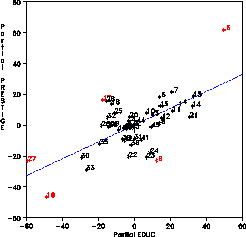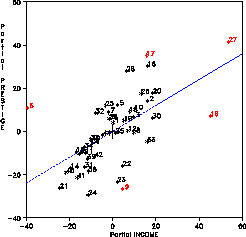partial partial - Macro for partial regression residual plots partial

# SAS Macro Programs: partial

\$Version: 1.8-1 (30 Nov 2007)
Michael Friendly
York University

## PARTIAL macro (get partial.sas)

The PARTIAL macro draws partial regression plots as described in "Section 5.5". These are high-resolution versions of the plots produced by the PARTIAL option of the REG procedure, with options for labelling influential points.

Version 1.6 of the macro has been made more computationally efficient following (Velleman and Welsch, 1981), allows SAS shorthand notation (X1-X5, AGE--WEIGHT, or _NUMERIC_) for the list of predictors in the XVAR= option, and also provides partial residual plots ("added-variable" or "component-plus-residual" plots), as specified by the TYPE= parameter.

### Parameters

DATA = _LAST_
Name of the input data set. If not specified, the most recently created data set is used.
YVAR =
Name of the dependent variable in the model.
XVAR =
List of independent variables in the model. The list of variables may be given explicitly or using the range notation X1-X n.
ID =
Name of an optional character or numeric variable used to label observations. If ID= is not specified, the observations are identified by the numbers 1, 2, ...
LABEL=INFL
Specifies which points in the plot should be labelled with the value of the ID= variable. If LABEL=NONE, no points are labelled; if LABEL=ALL, all points are labelled; otherwise (LABEL=INFL) only potentially influential observations (those with large leverage values or large studentized residuals) are labelled.
OUT =
Name of the output data set containing partial residuals. This data set contains ( p + 1 ) pairs of variables, where p is the number of XVAR= variables. The partial residuals for the intercept are named UINTCEPT and VINTCEPT. If XVAR=X1 X2 X3, the partial residuals for X1 are named UX1 and VX1, and so on. In each pair, the U variable contains the partial residuals for the independent (X) variable, and the V variable contains the partial residuals for the dependent (Y) variable.
HTEXT
Specifies the height of text labels in the plots.
PLOTS=&XVAR
Specifies which partial plots to produce. This can be a subset of the XVAR variables, or INTCEPT &XVAR to include the partial plot for the intercept, or NONE if no plots are desired.
TYPE=PARTREG
TYPE=PARTREG (the default) produces partial regression plots, in which all other variables are partialled from both the Y and X variables. TYPE=PARTRES produces partial residual plots.
GOUT=GSEG
Name of graphic catalog used to store the graphs for later replay.
NAME=PARTIAL
The name assigned to the graphs in the graphic catalog.

### Computing note

In order to follow the description in the text, the program computes one regression analysis for each regressor variable (including the intercept). Velleman & Welsh (1981) show how the partial regression residuals and other regression diagnostics can be computed more eficiently--from the results of a single regression using all predictors. They give an outline of the computations in the PROC MATRIX language.

### Usage Note

When using the PARTIAL macro with the SAS System for Personal Computers, it may be neccessary to add the option WORKSIZE=100 to the PROC IML statement.

### Example

This example produces partial residual plots for the Duncan data on the relation between occupational prestige and income and education. These statements produce the figures below:
```title h=1.5 'Partial regression residuals';
%include data(duncan);

%partial(data=duncan,
yvar=prestige,xvar=educ income,
id=case,label=ALL);
```
Two plots are produced. All obsservations are labelled with their CASE number; observations which are eitherinfluential, or high-leverage points are drawn in red.